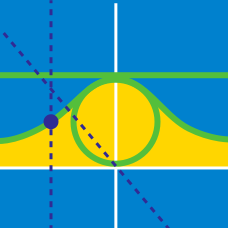Calculus

# Parametric Equations - Surface Area

What is the surface area $S$ of the body of revolution obtained by rotating the curve $y=e^x,$ $0 \le x \le 1,$ about the $x-$axis?

What is the surface area $S$ of the body of revolution obtained by rotating the parametric curve $\begin{array}{c}&x = 8 t^2 + 9 &y = -4 t &0 \leq t \leq 1 \end{array}$ about the $x$-axis?

What is the surface area $S$ of the solid of revolution obtained by rotating the parametric curve $\begin{array}{c}&x = 4 t &y = 6 t &0 \leq t \leq 2 \end{array}$ about the $y$-axis?

What is the surface area $S$ of the body of revolution obtained by rotating the parametric curve $\begin{array}{c}&x = 7 \cos t &y = 7 \sin t &0 \leq t \leq \pi \end{array}$ about the $x$-axis?

If $S$ is the surface area of the solid obtained by rotating the parametric curve $\begin{array}{c}&x = 4 \cos^3 t &y = 4 \sin^3 t &0 \leq t \leq \frac{\pi}{2} \end{array}$ about the $x$-axis, what is $\frac{5}{2}S?$

×